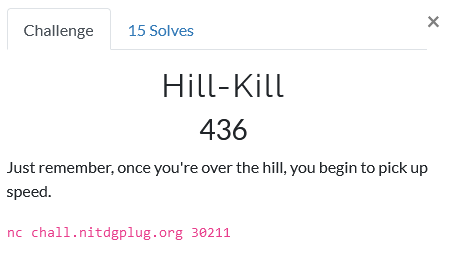# Foobar CTF 2021 - Hill-Kill

Cryptography – 436 pts (15 solves)

Iterative Hill cipher server challenge, given ciphertext and key.

## ChallengeHill-Kill, is that the newest Tarentino movie? Dad jokes aside, this challenge title is quite telling: Hill cipher!. It’s a nice cipher based on linear algebra, so some knowledge of that might be helpful for solving this challenge.

Upon connection we are given the key and a ciphertext, so this should be quite easy. Perhaps there is some sneaky trick or complication that might show up, but for now it seems quite straightforward… So let’s get crackin’!

## Solution

How does one kill a hill? With its key of course, its decryption key to be specific. The server gives us, what I assumed to be, the encryption key. So we need to invert that, but how? As I mentioned before, the Hill cipher uses linear algebra and a simple numerical mapping (A-Z -> 0-25) to encrypt plain text as follows

cipher vector (Nx1) = encryption key matrix (NxN) * plain vector (Nx1)


where both the full cipher and plain text are divided up into vectors of size N, the key matrix is square and should be invertible (!), and * represents matrix multiplication.

Now that we know how the encryption work, what about decryption? Well, using the law of linear algebra we can simply reverse the operation as

cipher vector (Nx1) * inverse( encryption key matrix (NxN) ) = plain vector (Nx1)


where we have inversed the encryption key matrix. Matrix inversion is a specific operation that is only allowed on square matrices with a non-zero determinant. To not complicate things too much, I simply rely on the server to give valid encryption keys. Thus to get the decryption matrix we follow these steps:

1. Convert the received key string (length N**2) into an encryption matrix (size NxN).
2. Numerically inversing the encryption matrix using the numpy.linalg module.
3. Scale the inverse matrix by d * modinv(d), where d is the determinant of the encryption matrix.
4. Round off all entries to integers and take modulo 26 (not 27!).

For those unknown to the ways of linear algebra this may seem like magic. I used to teach linear algebra (for physics) at my university, so hit me up if you have any questions :). Alternatively, there are many great sources on the internet (esp. 3Blue1Brown on YoutTube).

Now the only thing that rests us to do is to multiply our decryption matrix with the plain text vectors, again modulo 26, and send them to the server. In order to kill all the hills that stood in my way, I used the script below. >:)

#!/usr/bin/env python3

# Imports
from pwn import *
import numpy as np
import numpy.linalg as la

# Connection
host = "chall.nitdgplug.org"
port = 30211

context.log_level = "debug"

s = remote(host, port)

# Used alphabet
alp = "abcdefghijklmnopqrstuvwxyz"

# Loop to kill all the hills >:)
while True:

# Retrieve the key and ciphertext
_, key, ct, _ = s.recv().decode('ascii').split('\n')

key = key.split(': ')[-1]
ct  = ct.split(': ')[-1]

# Visual check
print(key)
print(ct)

# Extract key size (NxN) from N**2 length key
key_size = int(np.sqrt(len(key)))
#print(key_size, key_size)

# Construct the key array (size NxN)
enc_keyarr = np.array([alp.index(i) for i in list(key)]).reshape(key_size, key_size)
#print(enc_keyarr)

# Construct the cipher text vectors (size Nx1)
ctvec = np.array([alp.index(i) for i in list(ct)])
ctarr = np.array([list(ctvec)[i:i+key_size] for i in range(0,len(ct),key_size)])
#print(ctarr)

# Get key array determinant
keyarr_det = int(round(la.det(enc_keyarr)))

# Construct decryption key array in a nice format :)
dec_keyarr = np.round(( la.inv(enc_keyarr) * keyarr_det * pow(keyarr_det, -1, 26) ) % 26 ).astype(int)
#print(dec_keyarr)

# Multiple the decryption key array with each cipher text vector to obtain the message vectors
mvec = [np.dot(dec_keyarr, vec) % 26 for vec in ctarr]
#print(mvec)

# Construct the answer from msg vecs
ans = ''
for vec in mvec:
for val in vec:
ans += alp[val]

# Visual check
print(ans)

# Send the answer to the server
s.sendline(ans)


Ta-da!

GLUG{17_15_34513r_70_g0_d0wn_4_h1ll_7h4n_up_bu7_7h3_v13w _15_fr0m_7h3_70p}